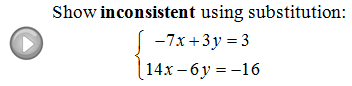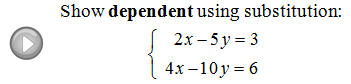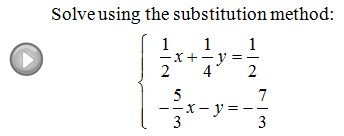## Pages

Showing posts with label Linear equation. Show all posts
Showing posts with label Linear equation. Show all posts

### Solving Linear Systems by Substitution

The substitution method for solving linear systems is a completely algebraic technique.  There is no need to graph the lines unless you are asked to.  This method is fairly straight forward and always works, the steps are listed below.

Solve the system using the substitution method:
Step 1: Use either equation and solve for a variable.
In this case, we solved the first equation for y.
Step 2: Substitute the resulting quantity into the other equation.
Here we substituted  the quantity found for y into the second equation.
Step 3: Solve for the remaining variable.
Step 4: Back substitute to find the value for the other variable.
Step 5: Present your answer as an ordered pair (x, y).
It does not matter if you choose to solve for x or y first. However, make sure that you do not substitute into the same equation in step 2.

Remember that we are trying to find the simultaneous solutions or the points where the two lines intersect.  Next we will see what happens when the system is dependent, in other words, when the system consists of two lines that are the same.

Solve the system using the substitution method:
Solve for y in the first equation.

Any true statement, including 0 = 0, indicates a dependent system.
The next system consists of two parallel lines which has no simultaneous solution.

Solve the system using the substitution method:
Solve for x in the first equation.
Any false statement indicates an inconsistent system.

Solve the systems using the substitution method.
Typical word problem: When Joe walked away from the craps table he had 45 chips. He had a combination of \$5 and \$25 chips that added to a total of \$625.  How many of each chip did he have?
Set up a system of two linear equations.

Solve the system.
Answer: Joe had 25 five-dollar chips and 20 twenty-five dollar chips.

Video Examples on YouTube:### Solving Linear Systems by Graphing

The systems in this section will consist of two linear equations and two unknowns  Given linear equations, we are asked to find out if they have simultaneous solutions.  In other words, where do the two lines intersect?  This question brings up three cases:
Most of the time the linear system will have a common point, (x, y). The point where they cross is the solution to the system.
However, not all linear systems have on ordered pair solution; some have no common points and others have infinitely many.  Imagine that you were asked to solve the system consisting of two parallel lines, where do they cross?  In this case, there is no simultaneous solution and the system of two parallel lines is inconsistent. In the case where the system consists of two lines that happen to be the same line, there are infinitely many common points.  This system is dependent and solutions can be presented in the form (x, y) where x can be any real number and y = mx + b.

Solve the systems given the graph:
Is the ordered pair a solution to the system?
The next example shows how to find the common point, the point where the two lines intersect, if we are given a linear system in standard form.

Solve the system:
Step 1: Place the linear equations in slope-intercept form.
Step 2: Graph the lines and use the graph to find the common point.
Step 3: Check your answer and present it as an ordered pair.
Accuracy here is important, use graph paper and a straight edge when using the graphing method to solve linear systems.

Solve the system using the graphing method:
Graph the lines using slope-intercept form.

Solve the system using the graphing method:
Graph the lines using slope-intercept form.
Take extra care with the scale when graphing to find the intersection.  Make sure all your tick marks are equal in size.  This will make your graphs more accurate and easier to read.  Check to see that your answer works for BOTH equations.

Solve the system using the graphing method:
Place lines in slope-intercept form.
And then graph them.
Solve the system using the graphing method:
Place lines in slope-intercept form and then graph them.
Dependent systems seem to give beginning algebra students the most trouble.  Remember that we are looking for points where the two lines intersect.  If the lines are the same, well then they will cross at infinitely many points.  Because of this we have to use special notation to indicate an infinite set.  Notice that we have already put the line in y-intercept form, y = mx + b, so it is not a big leap to write the set final ordered pair solutions in the form (x, mx + b).
You might see different notation in other texts such as,
Video Examples on YouTube: